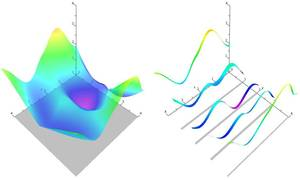# Practical Mixed-Integer Optimization for Geometry Processing

David Bommes, Henrik Zimmer, Leif Kobbelt
Special Issue of Lecture Notes in Computer Science 2012
Proceedings of Curves and Surfaces 2010Solving mixed-integer problems, i.e., optimization problems where some of the unknowns are continuous while others are discrete, is NP-hard. Unfortunately, real-world problems like e.g., quadrangular remeshing usually have a large number of unknowns such that exact methods become unfeasible. In this article we present a greedy strategy to rapidly approximate the solution of large quadratic mixed-integer problems within a practically sufficient accuracy. The algorithm, which is freely available as an open source library implemented in C++, determines the values of the discrete variables by successively solving relaxed problems. Additionally the specification of arbitrary linear equality constraints which typically arise as side conditions of the optimization problem is possible. The performance of the base algorithm is strongly improved by two novel extensions which are (1) simultaneously estimating sets of discrete variables which do not interfere and (2) a fill-in reducing reordering of the constraints. Exemplarily the solver is applied to the problem of quadrilateral surface remeshing, enabling a great flexibility by supporting different types of user guidance within a real-time modeling framework for input surfaces of moderate complexity.